In this mini-lesson, we will explore the world of zeros of a quadratic polynomial.

We will try to understand the methods to find the zeros or roots of a quadratic polynomial, observe the roots graph, and discover other interesting aspects of it.

You can check out the interactive simulations to know more about the lesson and try your hand at solving a few interesting practice questions at the end of the page.

Before we get started, let's have a look at a simple question.

Have you ever observed the path of a ball when it is thrown in the air?Its trajectory can be modeled by a quadratic polynomial.

The word "Quadratic" is derived from the word "Quad" which means square.

In other words, a quadratic polynomial is a “polynomial function of degree 2”

There are many scenarios where quadratic polynomials are used.

In fact, when a rocket is launched, its path is described by the zero of a quadratic polynomial.

## Lesson Plan

 1 What Do You Mean By Zeros of a Quadratic Polynomial? 2 Important Notes on Zeros of a Quadratic Polynomial 3 Solved Examples on Zeros of a Quadratic Polynomial 4 Thinking Out of the Box! 5 Interactive Questions on Zeros of a Quadratic Polynomial

## What Do You Mean By Zeros of a Quadratic Polynomial?

A polynomial of the form $$p(x)=ax^2+bx+c$$, where $$a \neq 0$$ is called a quadratic polynomial.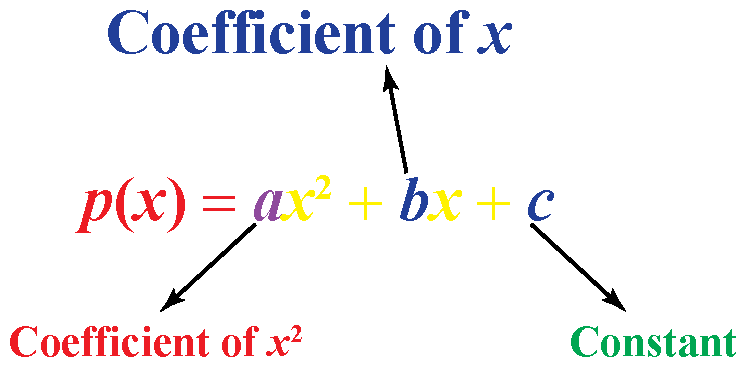### Zeros of Quadratic Polynomial: Definition

real number $$"k"$$ of a quadratic polynomial $$p(x)$$ is 0 if $$p(k)=0$$ .

Consider the polynomial: $$p(x)=x^2-3x-4$$

Let's find the value of the polynomial at $$x=-1$$ by substituting -1 for $$x$$ in $$p(x)$$.

\begin{align}p(-1)&=(-1)^2-3(-1)-4\\&=1+3-4\\&=0\end{align}

So, here $$x=-1$$ is a zero of the quadratic polynomial $$p(x)=x^2-3x-4$$.

## How To Find Zeros of a Quadratic Polynomial?

There are two methods to find the zeros of a quadratic polynomial:

Let us consider the quadratic polynomial $$2x^2+x-3=0$$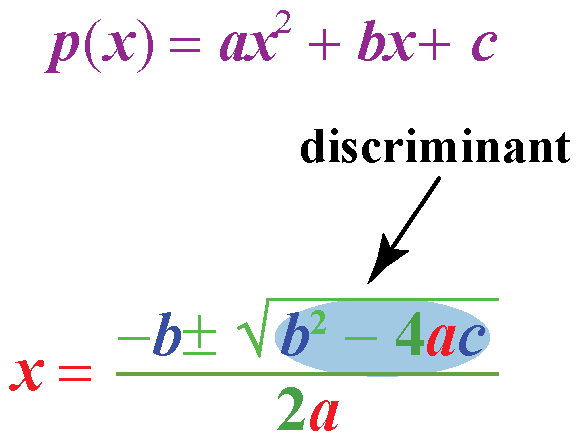Let’s find the determinant to find the nature of the solution.

\begin{align}b^2-4ac&=1^2-4\times 2\times (-3)\\&=1+24\\&=25>0\end{align}

This means that the quadratic polynomial has two real and distinct zeros.

Just plug in the values $$a=2$$, $$b=1$$ and $$c=-3$$ in the quadratic formula.

\begin{align}x&=\frac{-b\pm \sqrt{b^2-4ac}}{2a}\\&=\frac{-1\pm \sqrt{1^2-4\times 2\times (-3)}}{2\times2}\\&=\frac{-1\pm \sqrt{25}}{4}\\&=\frac{-1\pm 5}{4}\\&=-\frac{3}{2},1\end{align}

 \begin{align} x=-\frac{3}{2},1 \end{align}

### Factorization

Let’s solve this quadratic polynomial by factorization method.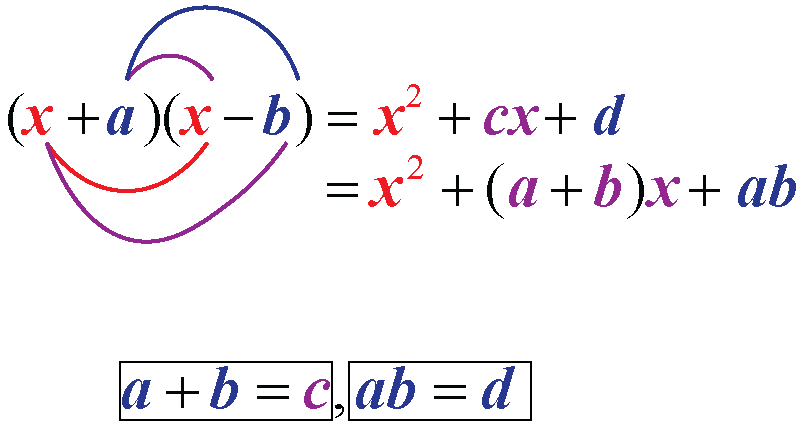Here, we need to find $$a$$ and $$b$$ such that $$a+b=1$$ and $$ab=-6$$

Considering this, we see that the numbers are $$a=3$$ and $$b=-2$$
\begin{align}2x^2+x-3&=0\\2x^2+3x-2x-3&=0\end{align}

Take the common factors out from the first two terms and last two terms.

So, this quadratic polynomial factoring gives:
\begin{align}x(2x+3)-(2x+3)&=0\2x+3)(x-1)&=0\end{align} When the product of two numbers is \(0, then either of the numbers are $$0$$

This means $$2x+3=0$$ or $$x-1=0$$

Solving these two equations gives

 \begin{align} x=-\frac{3}{2},1 \end{align}

## Sum and Product of Zeros of Quadratic Polynomial

Let's understand the relationship between zeros and coefficients of a quadratic polynomial.

If $$\alpha$$ and $$\beta$$ are zeros of a quadratic polynomial, $$x^2+bx+c=0$$, the sum of zeros is equal to the negative of $$b$$ and the product of zeros is equal to the constant term $$c$$.

Mathematically, this forms the following two equations:

 $$\alpha + \beta = -b,\;\;\; \alpha \cdot \beta =c$$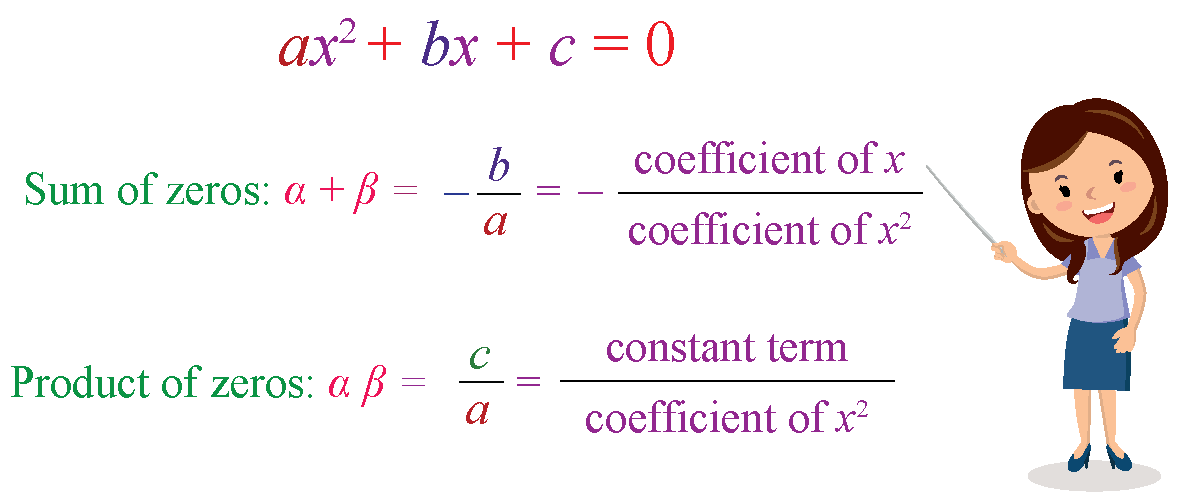## Representing Zeros On Graph

Now, you know that a real number $$k$$ is a zero of a quadratic polynomial $$p(x)$$ if $$p(k)=0$$.

Let us see the geometrical meaning of the zeros of a quadratic polynomial.

Consider the quadratic polynomial $$2x^2+x-3=0$$

This is how the quadratic polynomial is represented on a graph.

This curve is called a parabola.

### Roots GraphThe zeros of the quadratic polynomial $$2x^2+x-3=0$$ are the $$x$$ coordinates of the points where the graph of polynomial intersects the $$x$$-axis.

So, the zeros of the quadratic polynomial $$2x^2+x-3=0$$ are $$-\dfrac{3}{2}$$ and 1

Here is quadratic roots calculator for you to find the zeros of a quadratic polynomial using a graph.

Use the simulation below by dragging the values of $$a$$, $$b$$, and $$c$$ to experiment with different graphs.

The points where the curve intersects the $$x$$-axis are the roots of the zeros of the quadratic polynomial.

## Solved Examples

 Example 1

Ellie knows that the zeros of a quadratic polynomial are -2 and -7Can you find the quadratic polynomial whose zeros are -2 and -7?

### Solution

The zeros of the quadratic polynomial are -2 and -7

Let $$a=-2$$ and $$b=-7$$.

Then, $$a+b=-9$$ and $$a\times b=14$$

So, if $$a$$ and $$b$$ are the zeros of the quadratic polynomial, the quadratic polynomial is given by $$x^2-(a+b)x+ab$$.

\begin{align}x^2-(a+b)x+ab&=x^2-(-9)x+14\\&=x^2+9x+14\end{align}

 So, the quadratic polynomial is $$x^2+9x+14$$.
 Example 2

Find the zeros of the quadratic polynomial $$p(x)=x^2-5$$ and verify the relationship between the coefficients of the polynomial and its zeros.

Solution

Recall the algebraic identity: $$a^-b^2=(a+b)(a-b)$$

Using this identity, we get $$p(x)=x^2-5=(x+\sqrt{5})(x-\sqrt{5})$$.

So, the value of polynomial is zero when $$x=\sqrt{5},-\sqrt{5}$$.

These are the roots of quadratic polynomial.

\begin{align}\text{Sum of zeros}&=\sqrt{5}-\sqrt{5}\\&=0\\&=-\dfrac{\text{Coefficient of $$x$$}}{\text{Coefficient of $$x^2$$}}\end{align}

\begin{align}\text{Product of zeros}&=-\sqrt{5}\times\sqrt{5}\\&=-5\\&=-\dfrac{5}{1}\\&=-\dfrac{\text{Constant term}}{\text{Coefficient of $$x^2$$}}\end{align}

 So, the zeros are $$x=\sqrt{5},-\sqrt{5}$$ and the required relationship is verified.
 Example 3

Mia is a fitness enthusiast who goes running every morning.

The park where she jogs is rectangular in shape and measures 12 feet by 8 feet.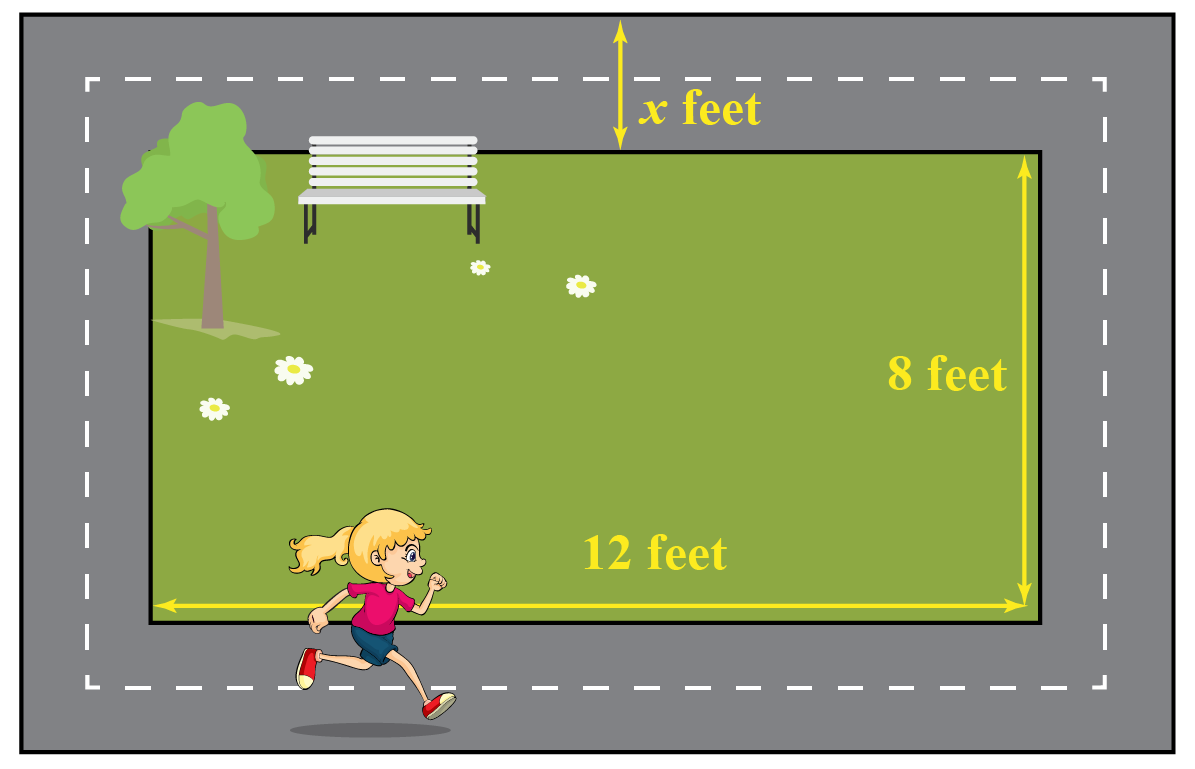A nature restoration group plans to revamp the park and decides to build a pathway surrounding the park.

This would increase the total area to 140 sq. ft.

What will be the width of the pathway?

Solution

Let’s denote the width of the pathway as $$x$$.

Then, the length and breadth of the outer rectangle is $$(12+2x)\;\text{ft.}$$ and $$(8+2x)\;\text{ft.}$$

The area of the park is:
\begin{align}(12+2x)(8+2x)&=140\\2(6+x)\cdot 2(4+x)&=140\x+6)(x+4)&=35\\x^2+10x-11&=0\end{align} Now, we have to find the zeros of the quadratic polynomial \(p(x)=x^2+10x-11.

\begin{align}p(x)&=0\\x^2+11x-x-11&=0\\x(x+11)-(x+11)&=0\x+11)(x-1)&=0\\x&=1,-11\end{align} Since length can’t be negative, we can only consider \(x=1.

 $$\therefore$$, The width of the pathway will be 1 feet.Think Tank
 1. Frank has a two-digit secret lucky number with him. He gives a few hints to his friend Joe to crack it.He says "If 1 and -2 are two zeros of $$x^3-4x^2-7x+10$$, then the lucky number is the remaining zero of the polynomial." Can you guess the lucky number?

## Interactive Questions

Here are a few activities for you to practice.

## Let's Summarize

The mini-lesson targeted the fascinating concept of zeros of a Quadratic Polynomial. The math journey around zeros of a Quadratic Polynomial starts with what a student already knows, and goes on to creatively crafting a fresh concept in the young minds. Done in a way that not only it is relatable and easy to grasp, but also will stay with them forever. Here lies the magic with Cuemath.

At Cuemath, our team of math experts is dedicated to making learning fun for our favorite readers, the students!

Through an interactive and engaging learning-teaching-learning approach, the teachers explore all angles of a topic.

Be it worksheets, online classes, doubt sessions, or any other form of relation, it’s the logical thinking and smart learning approach that we, at Cuemath, believe in.

## FAQs on Zeros of a Quadratic Polynomial

### 1. How do you find the zeros of a quadratic polynomial?

The zeros of a quadratic polynomial can found by using the quadratic formula and factorization method.

### 2. Can a quadratic polynomial have no zeros?

Yes, it is possible that a quadratic polynomial has no zeros in real numbers. For example, the polynomial $$p(x)=x^2+1$$ have no zeros in real numbers.

### 3. How many zeros does a quadratic polynomial have?

A quadratic polynomial has at most two zeros.

### 4. How do you find the zeros of a quadratic polynomial from the graph?

The zeros of the quadratic polynomial are the $$x$$ coordinates of the points where the graph of polynomial intersects the $$x$$-axis.

### 5. What are the zeros of a polynomial?

We say a real number $$k$$ is a zero of a polynomial $$p(x)$$, if $$p(k)=0$$.

### 6. What are real zeros on the graph?

The real zeros of the quadratic polynomial are the $$x$$ coordinates of the points where the graph of polynomial intersects the $$x$$-axis.

### 7. How do you find the maximum real zeros?

The maximum real zeros of $$n^{\text{th}}$$ degree polynomial is $$n$$.

### 8. How do you find the sum of zeros of a quadratic polynomial?

The sum of zeros of a quadratic polynomial $$p(x)=ax^2+bx+c$$ is the negative of the coefficient of $$x$$, that is, $$-b$$.

### 9. How do you find the product of zeros of a quadratic polynomial?

The product of zeros of a quadratic polynomial $$p(x)=ax^2+bx+c$$ is the constant term, that is, $$c$$.

More Important Topics
Numbers
Algebra
Geometry
Measurement
Money
Data
Trigonometry
Calculus
More Important Topics
Numbers
Algebra
Geometry
Measurement
Money
Data
Trigonometry
Calculus